sibuzwaW

2021-08-12

For the two circles in Figures (a), (b), and (c), find the total number of common tangents (internal and external).
a)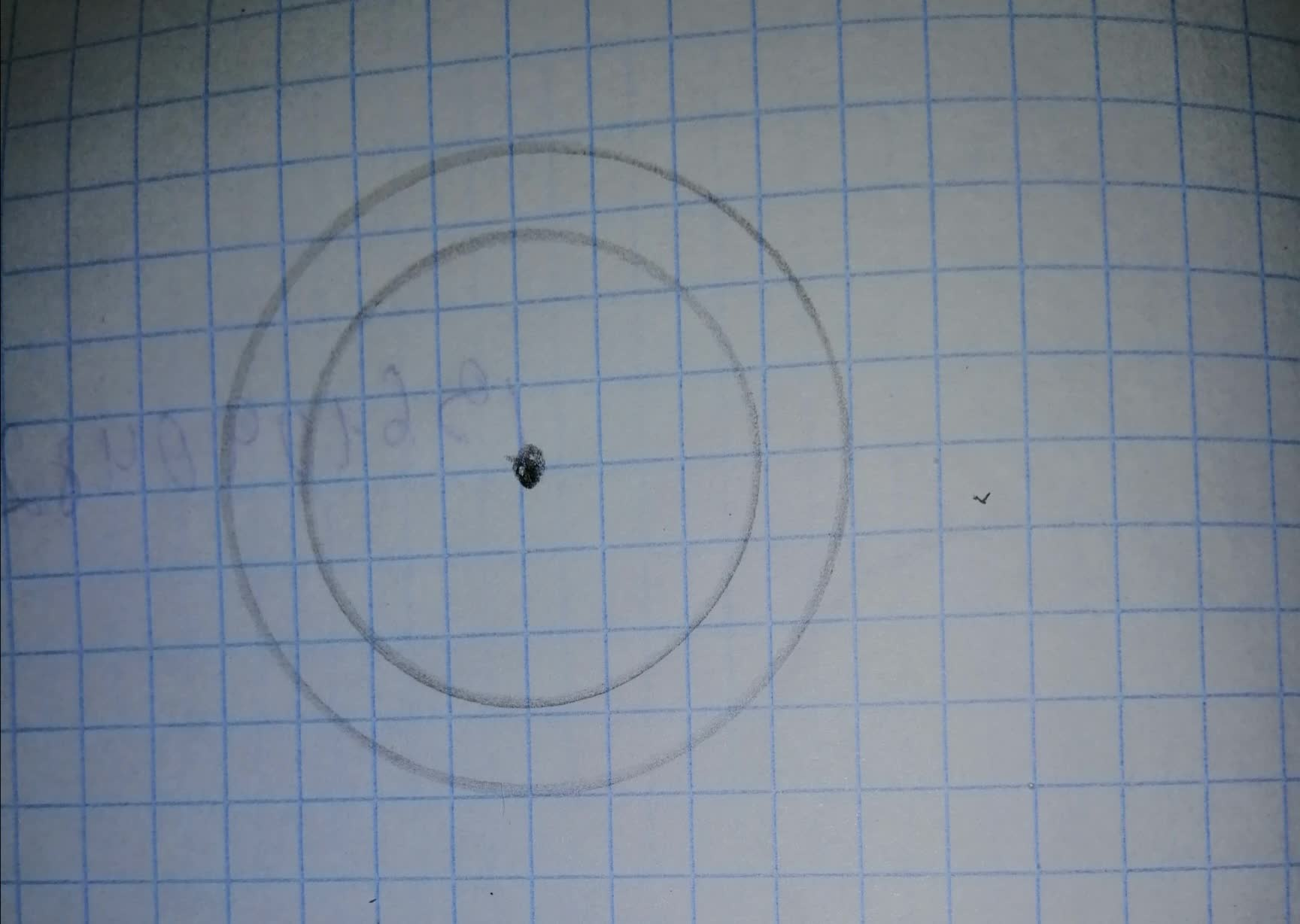b)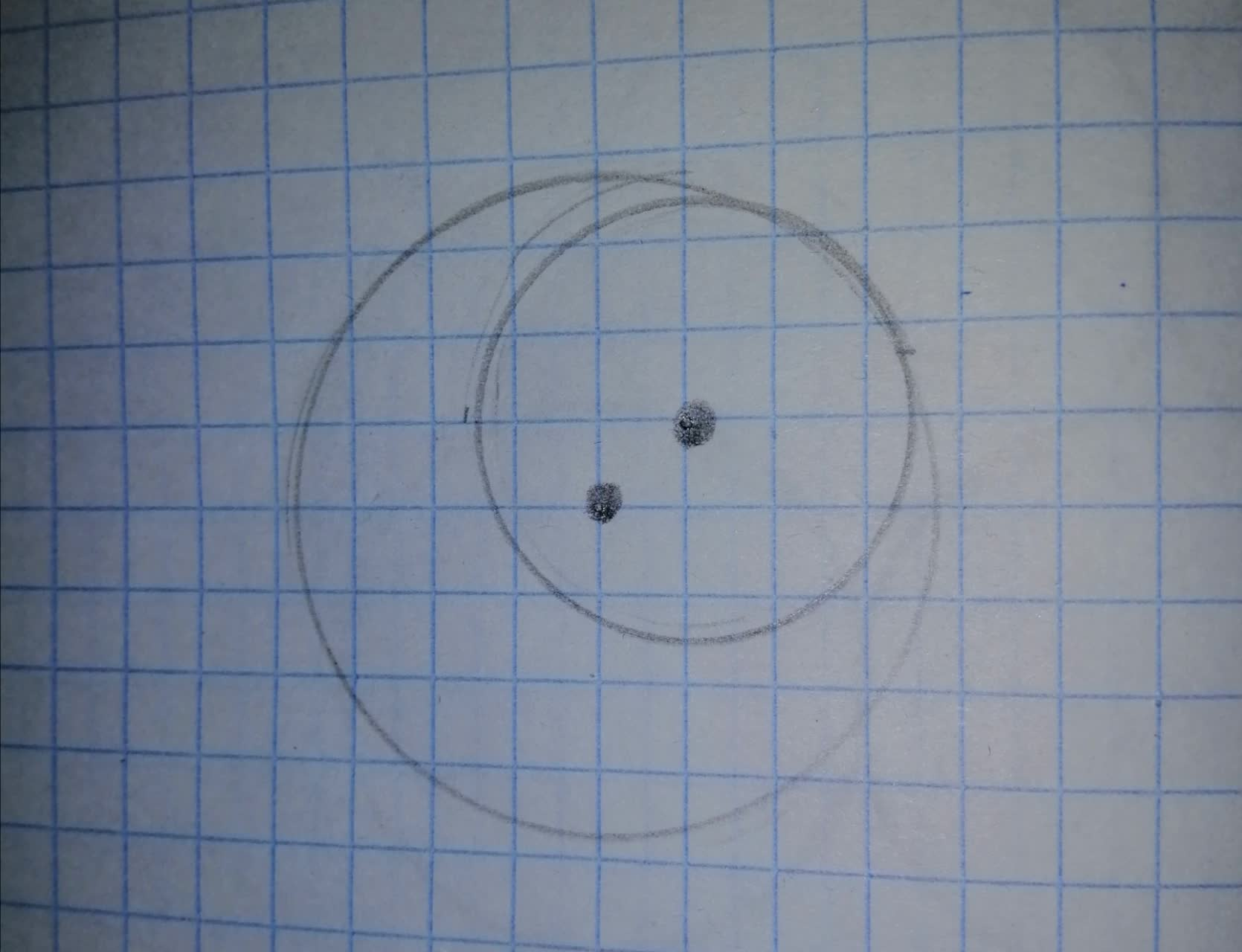c)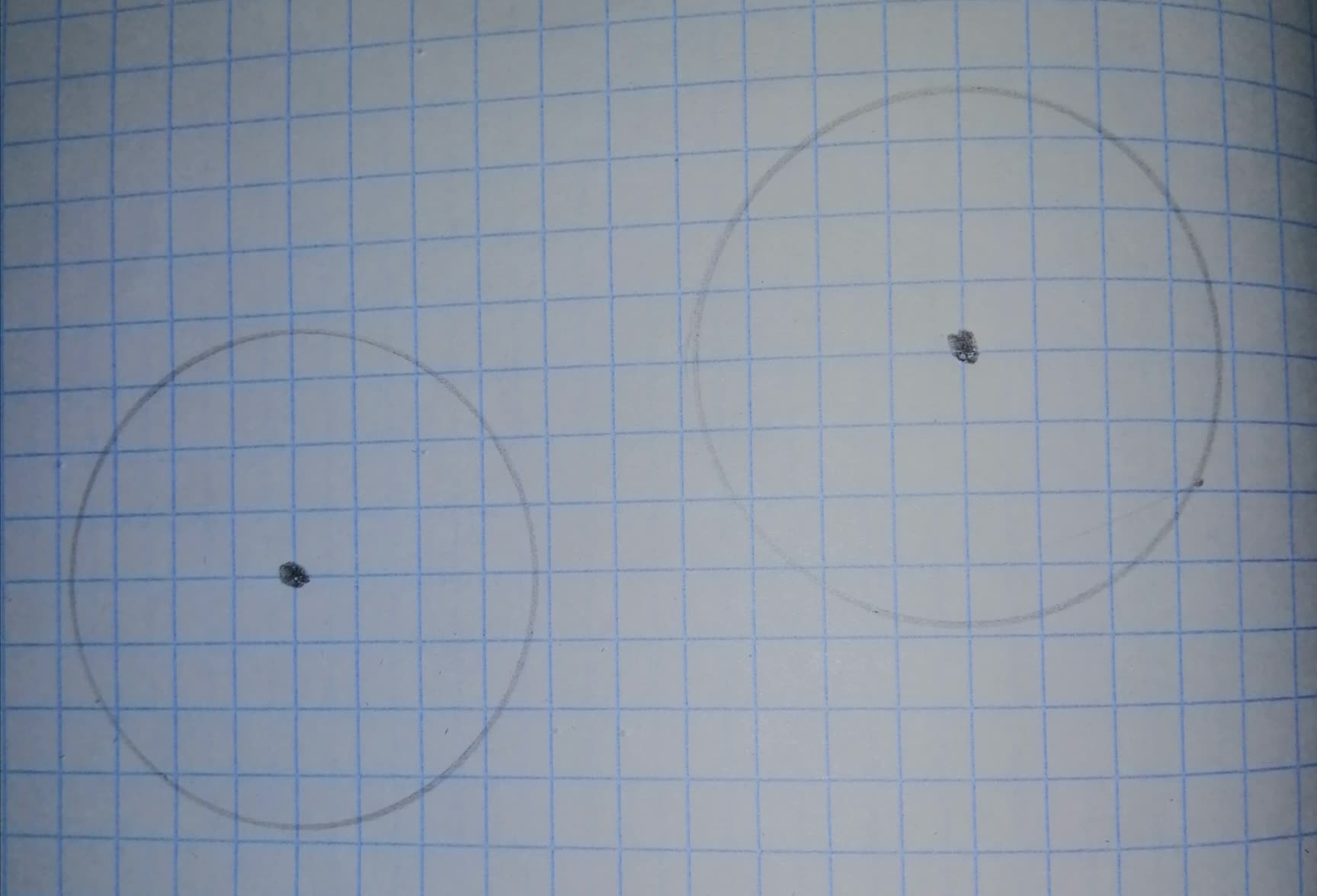Nathalie Redfern

Expert

Step 1
Consider the given figure (a),
in this case, one circle is lying inside another so there will be no common tangent.
because as any line touching the inner circle will always intersect the outer circle at two points as shown in the figure below,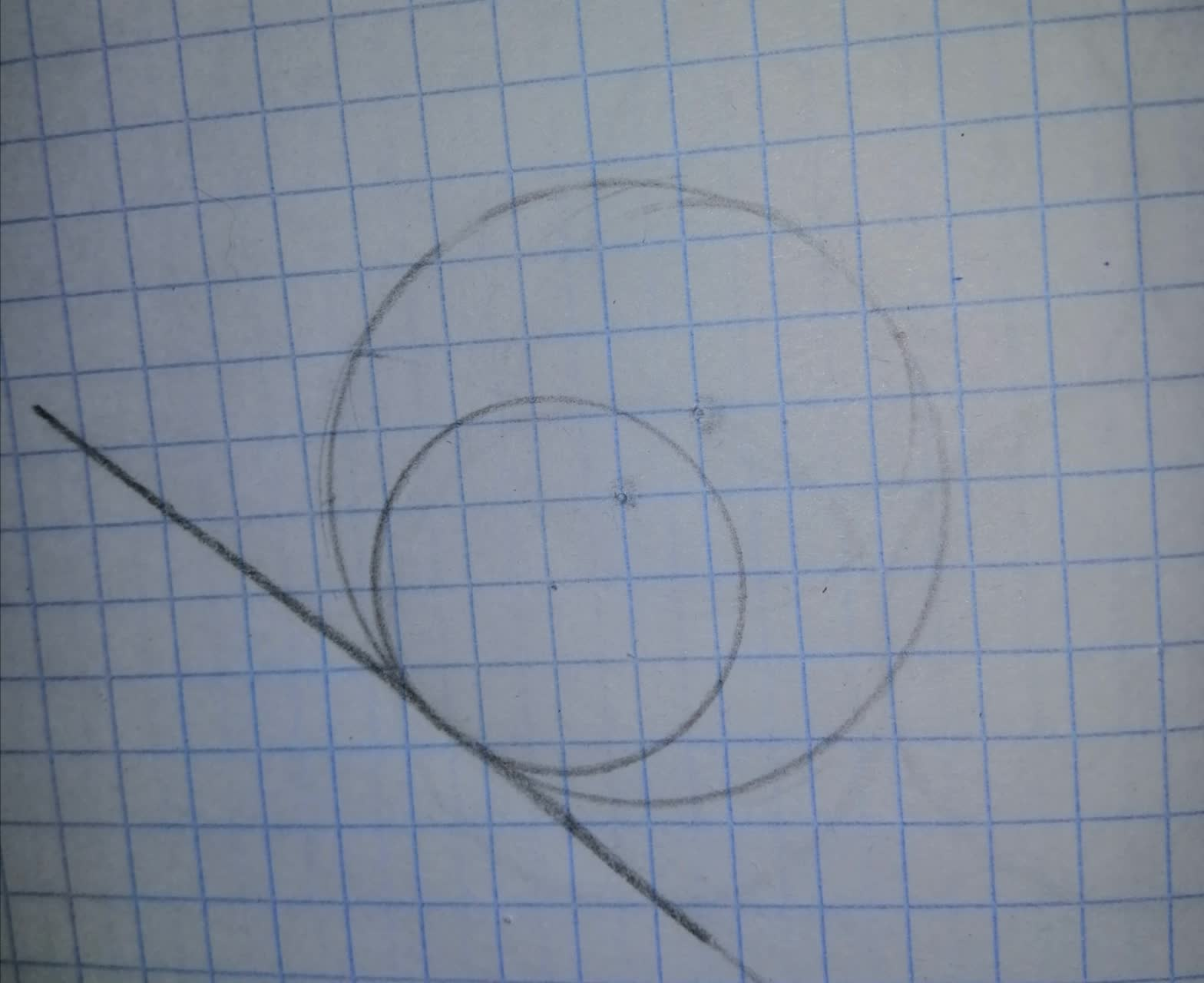Step 2
Consider the given figure (b),
In this case, both circles touching internally so there will be one common tangent.
as shown in the figure below,Step 3
Consider the given figure (c),
In this case, both circles lying outside of each other so there will be 4 common tangents.
there will be two transverse common tangents and two direct common tangents
as shown in the figure below,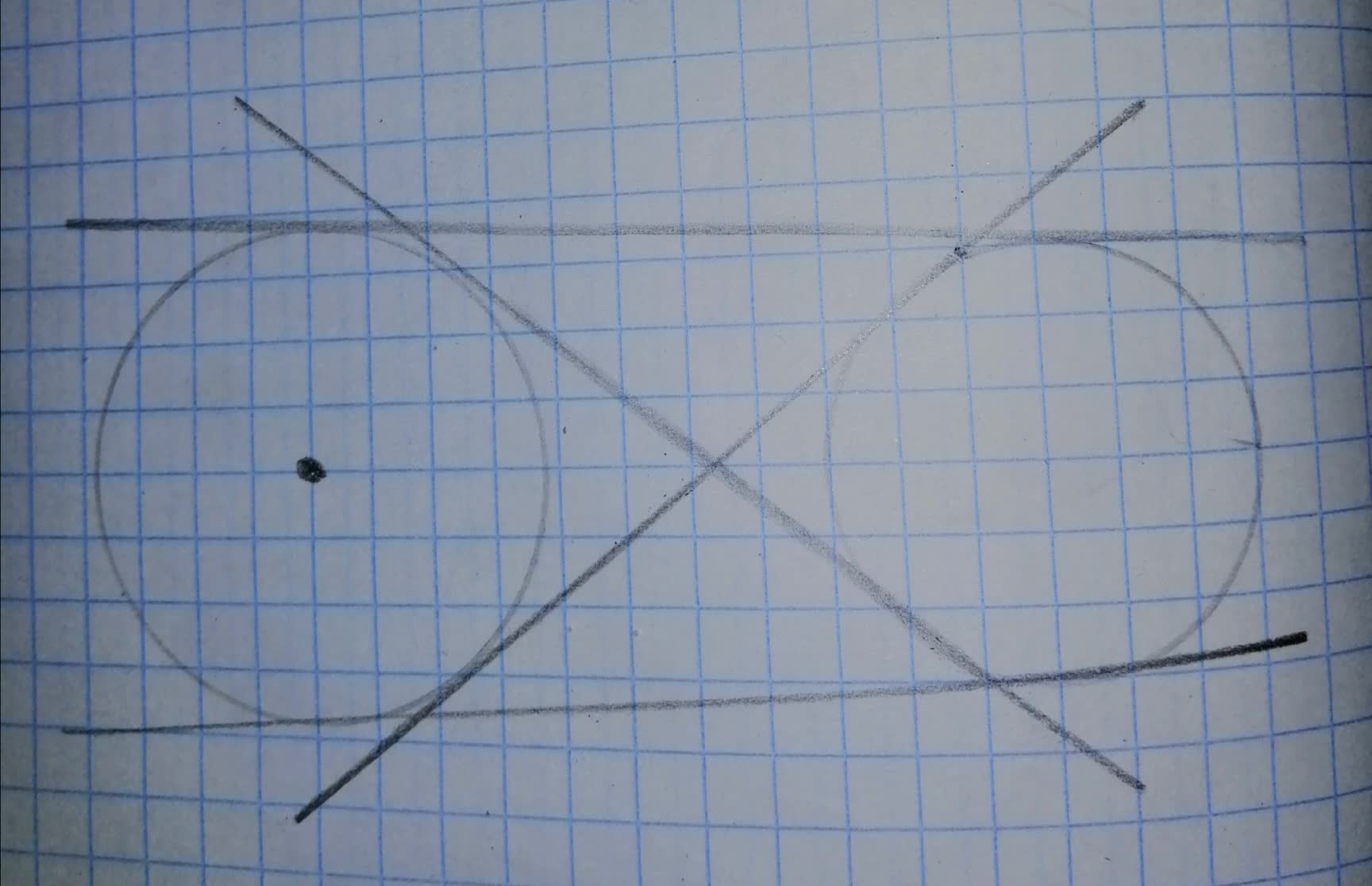Do you have a similar question?